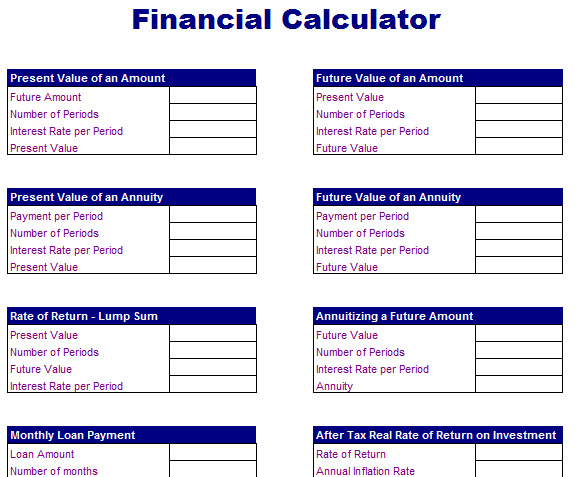# Basic Financial Calculator

Financial calculations is necessary for different parties such as owners of the organization, short and long term creditors, investors and the shareholders. There are four basic financial calculations for the business analysis such as payback period, NPV, IRR and ROI. These common calculations will help you to measure the impact of investment:

Payback Period: It is important to calculate the business because it is the time that a person will take to earn back the money spent on a project.

NPV: It is the present value of the future net cash flow of a project determined by applying a calculated discount rate.

IRR: It is a discount rate at which the NPV of a project equals to 0 and it represents the investment yield rate produced by the project.

ROI: The gain or loss of an asset is based on the investment or purchase price.

Common Financial Ratios

Some common financial ratios are calculated to compare financial status of the company to analyze the market trends and to take important decisions based on these calculations. Financial ratios are typically fall into three categories to provide very specific details. The first category includes “Liquidity Ratios” that specify the ability to meet its short-term obligations with some liquid assets. Second category includes “Financial Leverage Ratios” that will help you to evaluate the ability of a company to handle long-term responsibilities. Final category is known as “Profitability Ratios” that will help you to evaluate worth or financial value of your organization. Usually income statements, balance sheets and cash flow statements that will help you to calculate some important financial ratios.

Here is preview of a handy Basic Financial Calculator that can be very helping anywhere you got,Current Ratio

Current ratio is usually calculated to find the ratio of current assets and current liabilities.

Current Ratio = Current Assets/Current Liabilities

Quick Ratio

Quick ratio is also known as acid test ratio that is considered inflexible than the current ratio. In this ratio, inventories and paid expenses are removed from the current assets because these are not able to turn back into cash and not able to liquidate quickly.

Quick Ratio = (Cash + Investment + Receivables) / Current Liabilities

Liquidity Ratio

Liquidity ratio is more conservative ratio that helps you to evaluate the ability of the company to pay off all debts instantly on demand.

Liquidity Ratio = Cash + Marketable Securities / Current liabilities

Inventory Turnover Ratio

Inventory turnover ratio is calculated by dividing the cost of goods sold by the average value of inventories. This ratio will prove as a good indicator for the actual inventory liquidity.

• Inventory Turnover = Cost of Goods / Inventories
• Receivable Turnover = Sales on Credit / Accounts Receivable
• Financial Leverage Ratios
• Debt Ratio = Total Debt / Total Assets
• Debt to Equity Ratio = Total Debt / Total Equity
• Interest Earned Ratio = Earnings Before Interest (EBIT) / Interest Charges

Profitability Ratios

• Gross Profit Margin = (Sales – Cost of Goods Sold) / Sales
• Return on Investment = Net Income / Total Assets
• Return on Equity = Net Income / Owner’s Equity